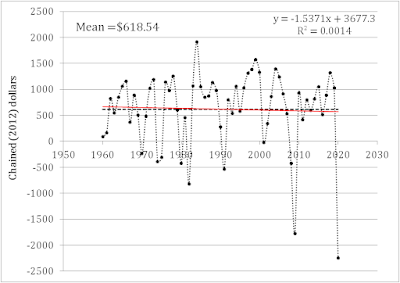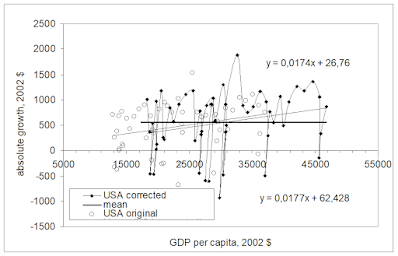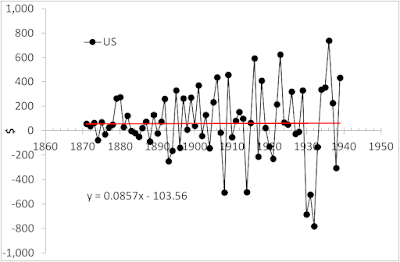## Real GDP Per Capita Growth: Any Blink By Blinken Will Be Considered As Fear

The evolution of the annual increment of the real GDP per capita in the USA can be represented as stochastic fluctuation around the mean value of \$648 between 1960 and 2020.Unsplash

This observation is presented in Figure 1, where the regression line of the annual increment (in chained (2012) dollars) is shown in red. The mean value is presented by a dashed line and highlights the negative slope of the regression line. This observation confirms our model of real GDP growth as introduced in 2004. The essence of this model can be formulated as follows:

There is no exponential economic growth in a capitalist economy and the return to capital is decreasing.

Therefore, a strong capitalist country has to rob all weaker countries.

In other words, the US (and other capitalist countries) future is grim because their prosperity depends on their capability to control and rob other countries. China and Russia make this route not easy to follow. It will be accompanied by extreme risks. The current US-Russia collision is just a start. China is a bigger challenge and any loss against Russia makes a tremendous crack in the NATO (West) defense.

Any blink by Blinken will be considered as fear and will destroy the belief in the US possibility to protect.Figure 1. Annual increment in real GDP per capita

The idea of constant annual increment of the real GDP per capita in developed counties was first introduced fifteen years ago in this working paper. I wrote “The trend has the simplest form – no change in absolute growth (annual increment) values and is expressed by the following relationship:

dG/dt=A (1)

where G is the absolute value of real GDP per capita, A is a constant. The solution of this equation is as follows:

G(t)=At+B (2)

where B=G(t0), t0 is the starting time of the studied period. Hence, the evolution of real GDP per capita is represented by a straight line if the second factor of growth has no cumulative effect. As discussed below, only some developed countries are characterized by a significant influence of the second factor.

Then, the relative growth rate can be expressed by the following relationship:

dG/Gdt=A/G(t) (3)

Relationship (3) indicates that the relative growth rate of per capita GDP is inversely proportional to the attained level of real GDP per capita, i.e. the observed growth rate should asymptotically decay to zero with increasing GDP per capita. “

Using (3) one can replace time, t, with G(t) and obtain the link between the G(t) and dG(t). For example, Figure 22 in this paper is copied here and presents the case of the US. The open circles are the estimates of real GDP per capita between 1950 and 2002. The regression line for the original data has a positive coefficient, i.e. the G(t) growth is slightly exponential.Figure 22 of the 2006 paper

In 2012, we revisited the model and re-estimated the annual increment in the USA. The Figure below is copied from the 2012 paper and includes data from 1950 to 2007, i.e. just before the Great Recession. The slope of the regression line is still positive. The Great Depression put the regression line to that observed in Figure 1 as we suggested in 2006.Figure 17 from the 2012 paper

Disclosure: None.